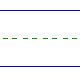Name: ______________________________________________ Date: _____________

Instructions: Find the sum.

 4 + 3 =6 + 3 =2 + 2 =4 + 1 =0 + 2 =0 + 0 =0 + 9 =6 + 0 =4 + 4 =1 + 8 =7 + 1 =7 + 2 =9 + 0 =0 + 8 =2 + 3 =3 + 1 =1 + 0 =8 + 0 =